# Gridded data:#

Plots of arrays and images $$Z_{i, j}$$ and fields $$U_{i, j}, V_{i, j}$$ on regular grids and corresponding coordinate grids $$X_{i,j}, Y_{i,j}$$.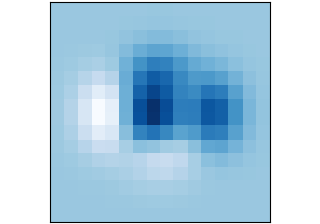imshow(Z)

imshow(Z)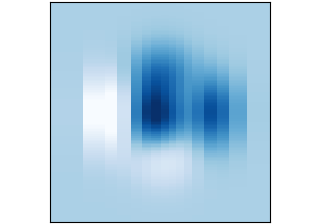pcolormesh(X, Y, Z)

pcolormesh(X, Y, Z)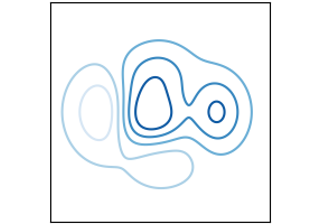contour(X, Y, Z)

contour(X, Y, Z)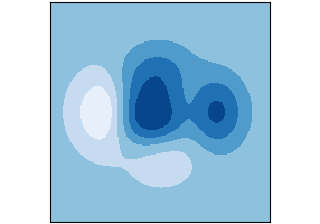contourf(X, Y, Z)

contourf(X, Y, Z)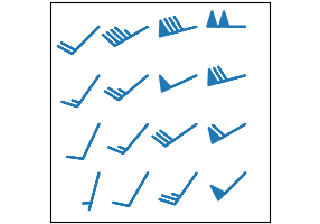barbs(X, Y, U, V)

barbs(X, Y, U, V)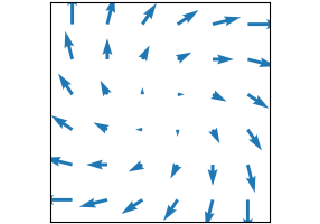quiver(X, Y, U, V)

quiver(X, Y, U, V)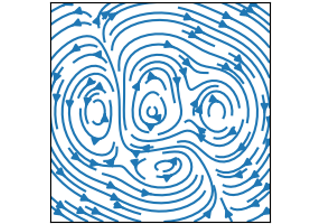streamplot(X, Y, U, V)

streamplot(X, Y, U, V)